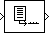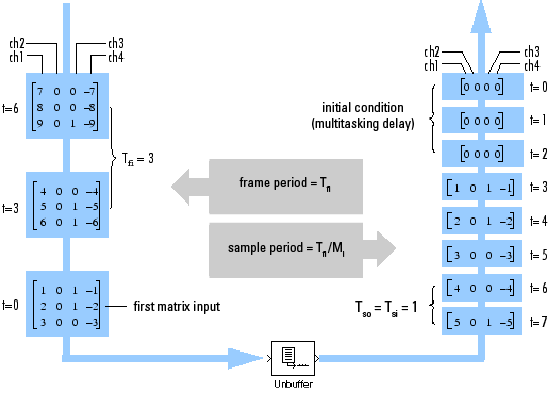# Unbuffer

Unbuffer input frame into sequence of scalar outputs

## Library

Signal Management / Buffers

`dspbuff3`

•## Description

The Unbuffer block unbuffers an Mi-by-N input into a `1`-by-N output. That is, inputs are unbuffered row-wise so that each matrix row becomes an independent time-sample in the output. The rate at which the block receives inputs is generally less than the rate at which the block produces outputs.The block adjusts the output rate so that the sample period is the same at both the input and output, Tso=Tsi. Therefore, the output sample period for an input of frame size Mi and frame period Tfi is Tfi/Mi, which represents a rate Mi times higher than the input frame rate. In the example above, the block receives inputs only once every three sample periods, but produces an output once every sample period. To rebuffer inputs to a larger or smaller frame size, use the Buffer block.

In the model below, the block unbuffers a four-channel input with a frame size of three. The Initial conditions parameter is set to zero and the tasking mode is set to multitasking, so the first three outputs are zero vectors.### Nonzero Latency

For multitasking operation, the Unbuffer block's buffer is initialized with the value specified by the Initial conditions parameter, and the block begins unbuffering this frame at the start of the simulation. Inputs to the block are therefore delayed by one buffer length, or Mi samples.

The Initial conditions parameter can be one of the following:

• A scalar to be repeated for the first Mi output samples of every channel

• A length-Mi vector containing the values of the first Mi output samples for every channel

• An Mi-by-N matrix containing the values of the first Mi output samples in each of N channels

Note

## Parameters

Initial conditions

The value of the block's initial output for cases of nonzero latency. You can specify a scalar, vector, or matrix.

## Supported Data Types

PortSupported Data Types

Input

• Double-precision floating point

• Single-precision floating point

• Fixed point (signed and unsigned)

• Boolean

• 8-, 16-, and 32-bit signed integers

• 8-, 16-, and 32-bit unsigned integers

Output

• Double-precision floating point

• Single-precision floating point

• Fixed point (signed and unsigned)

• Boolean

• 8-, 16-, and 32-bit signed integers

• 8-, 16-, and 32-bit unsigned integers

 Buffer DSP System Toolbox

See Unbuffer Frame Signals into Sample Signals for related information.

## Extended Capabilities

### Fixed-Point ConversionDesign and simulate fixed-point systems using Fixed-Point Designer™.

Introduced before R2006a

## Support